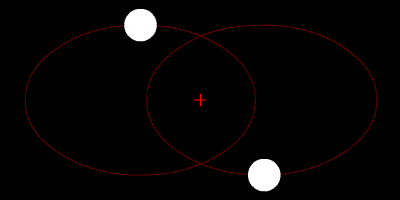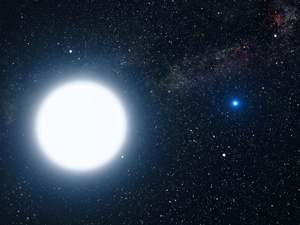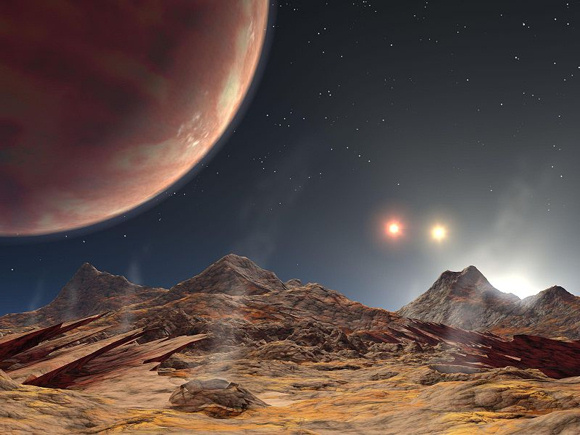## How astronomers learn masses of double stars

Astronomers observe double stars to find telltale clues to each star’s mass, and so get a gift from the heavens.A simulated example of a binary star system, whose component stars orbit around a common center of mass (red cross). In this depiction, the two stars have similar mass. In the case of the Sirius binary system, Sirius A has about twice the mass of Sirius B. Image via WikipediaAn artist’s impression of how Sirius A and B might appear to an interstellar visitor. Image credit: NASA, ESA and G. Bacon

Fortunately for astronomers, there are lots of binaries – two stars revolving around a common center of mass – populating the starry sky. A binary system is literally a gift from the heavens because it reveals to astronomers the masses of its two component stars.

To find the masses of stars in double systems, astronomers observe the mean distance between the two stars, often expressing the distance in astronomical units – the average distance between the Earth and sun. And they observe the time period for the two stars to revolve around one another, often expressed in Earth-years. With those two observations, they are able to calculate the stars’ masses, which they typically do in terms of solar masses*.

Perhaps half of the stars that we see at night are binaries. Take Sirius, for example, the brightest star of the nighttime sky. It looks like a single star to the unaided eye, but it’s actually a binary star. According to the star aficionado Jim Kaler, the two stars orbit each other in a period of 50.1 Earth-years at an average distance of 19.8 astronomical units. The brighter of the two is called Sirius A, while its fainter companion is known as Sirius B (The Pup).

So how would astronomers find the masses of Sirius A and B? They would simply plug in the observed distance separating the two component stars – and the orbital period – into the easy-to-use magical formula below.

Mass = a3/p2

In this formula, a is the mean distance between the stars (or, more precisely, the semi-major axis) in astronomical units, and p is the orbital period in Earth-years. So consider Sirius A and B with a separation of 19.8 astronomical units, an orbital period of 50.1 years.

Mass = a3/p2
Mass = 19.8 x 19.8 x 19.8/50.1 x 50.1
Mass = 7762.392/2510.01
Mass = 3.0925741 solar masses

The above answer gives the mass of both stars added together in solar masses. At this point, we know that the whole binary system equals about 3 solar masses, or is about 1,000,000 times more massive than our planet Earth. But we don’t yet know the masses of the individual stars Sirius A and Sirius B.

To find out the mass of each individual star, astronomers need to know the mean distance of each star from the barycenter – common center of mass. To learn this, once again they rely on their observations.

It turns out that Sirius B, the less massive star, is about twice as far from the barycenter (center of mass) than is Sirius A. That means Sirius B has about half the mass of Sirius A.

Thus, if you know the whole system is about three solar masses, you can deduce that the mass of Sirius A is about two solar masses, while Sirius B pretty much equals our sun in mass.

Nifty, yes?

*One solar mass is 1.9891 × 1030 kilograms about 333,000 times the mass of our planet Earth.Artist’s conception of the view from the (hypothetical) moon of the planet HD 188753 Ab (upper left) and the binary star HD 188753 BC over the moon’s horizon. Image credit: NASA

Bottom line: For astronomers, a binary or double star is a gift from the heavens. Astronomers observe these stars to find telltale clues to each star’s mass.

### Bruce McClure